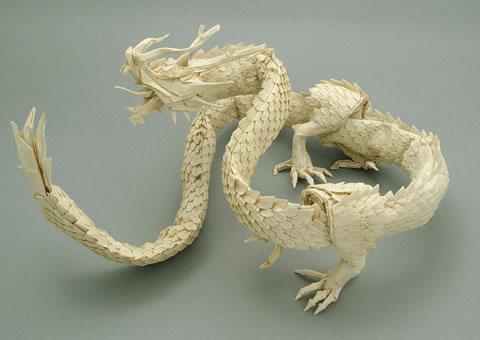# Boldly Folding (part 1)

Algebra Level 3You have a rectangular piece of paper of width $W$ and length $L$. You pick up the left side and fold it to the right side, like so:Which function describes the new position of a point $P = (x, y)$ on the paper after the fold?

1. f(x, y, W, L) = \left\{ \begin{array}{rl} (W-x,y) &\mbox{ if \$x<\frac{W}{2}\$} \\ (x, y) &\mbox{ otherwise} \end{array} \right.

2. f(x, y, W, L) = \left\{ \begin{array}{rl} (W^{2}-x,y+L-W) &\mbox{ if \$x>\frac{W}{2}\$} \\ (x, y) &\mbox{ otherwise} \end{array} \right.

3. $f(x, y, W, L) = (W - x, L-y)$

4. $f(x, y, W, L) = (\frac{\pi x}{W}, \frac{y}{\pi L})$

Details and assumptions:

• The paper has infinitesimal thickness.
×